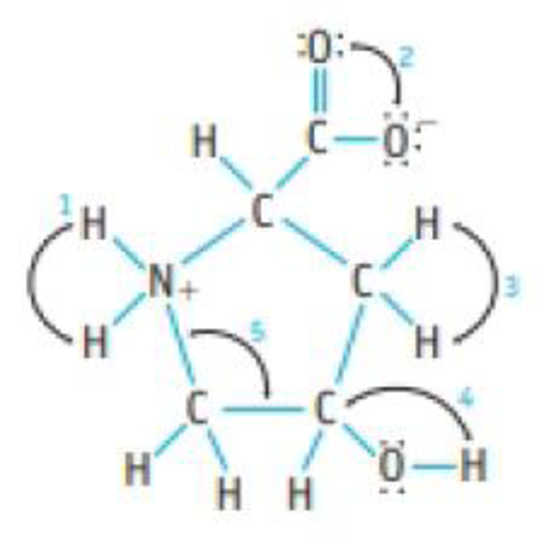Chapter 8, Problem 79GQ

Chapter
Section
Textbook Problem

Hydroxyproline is a less-common amino acid.(a) Give approximate values for the indicated bond angles. (b) Which are the most polar bonds in the molecule?

(a)

Interpretation Introduction

Interpretation: The bond angle in the given compound should be determined.

Concept Introduction:

Bond length: Bond distance or bond length is the average distance between nuclei of two bonded atoms in a molecule. It is a transferable property of a bond between atoms of fixed types

Bond angle: It is defined as the angle between the orbital contacting bonding electron pair around the central atom in a molecule ion. Bond angle is expressed in degree.

Explanation

The approximate values for the indicated bond angles are determined below:

Angle 1 = 1090 since there are four bond pairs and zero lone pairs around nitrogen atom hence geometry is tetrahedral.

Angle 2 = 1200 since there are three bond pairs and zero lone pairs around carbon atom hence geometry is trigonal planar

(b)

Interpretation Introduction

Interpretation: The most polar bond in the given compound should be determined.

Concept Introduction:

Bond length: Bond distance or bond length is the average distance between nuclei of two bonded atoms in a molecule. It is a transferable property of a bond between atoms of fixed types

Bond angle: It is defined as the angle between the orbital contacting bonding electron pair around the central atom in a molecule ion. Bond angle is expressed in degree.

Still sussing out bartleby?

Check out a sample textbook solution.

See a sample solution

The Solution to Your Study Problems

Bartleby provides explanations to thousands of textbook problems written by our experts, many with advanced degrees!

Get Started

What are the four main trophic levels?

Fundamentals of Physical Geography

In what ways is the celestial sphere a scientific model?

Horizons: Exploring the Universe (MindTap Course List)

What is population genetics?

Human Heredity: Principles and Issues (MindTap Course List)